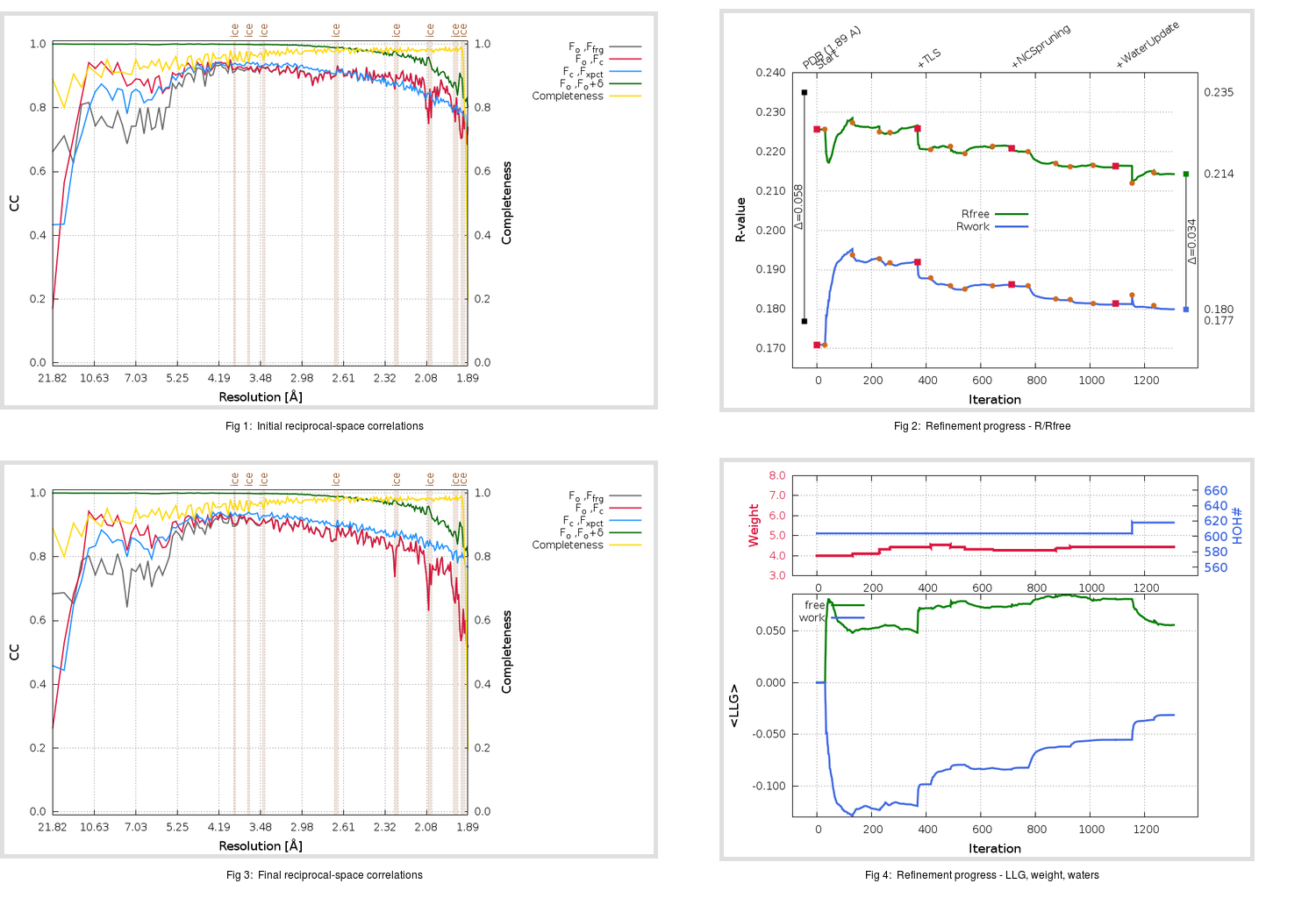Content:

## Deposited

` `
 Date deposited Date data collection Resolution R, Rfree 20110629 20091210 1.89 0.1740 0.2350

Molprobity (CCP4 7.0 version) summary:

```Ramachandran outliers =   0.49 %
favored =  96.22 %
Rotamer outliers      =   3.38 %
C-beta deviations     =     6
Clashscore            =   5.56
RMS(bonds)            =   0.0232
RMS(angles)           =   1.82
MolProbity score      =   1.96
Resolution            =   1.89
R-work                =   0.1740
R-free                =   0.2350
```

```Number of waters      =   604

<B> (all atoms) =   22.76 ( sd =   10.22 ) for       5410 non-hydrogen atoms
<B>   (protein) =   21.55 ( sd =    9.72 ) for       4780 non-hydrogen atoms
<B>     (water) =   31.89 ( sd =    9.33 ) for        604 non-hydrogen atoms
<B>    (others) =   31.17 ( sd =    6.65 ) for         26 non-hydrogen atoms

B min/max       (all non-hydrogen atoms) =    5.89 /   73.02
B min/max   (protein non-hydrogen atoms) =    5.89 /   73.02
B min/max     (water non-hydrogen atoms) =   10.37 /   55.69
B min/max     (other non-hydrogen atoms) =   14.23 /   40.79
```

## BUSTER (re-)refinement

` `

Molprobity (CCP4 7.0 version) summary:

```Ramachandran outliers =   0.16 %
favored =  97.53 %
Rotamer outliers      =   2.07 %
C-beta deviations     =     0
Clashscore            =   2.94
RMS(bonds)            =   0.0110
RMS(angles)           =   1.53
MolProbity score      =   1.42
Resolution            =   1.89
R-work                =   0.1800
R-free                =   0.2143
```

```Number of waters      =   618

<B> (all atoms) =   25.05 ( sd =   11.33 ) for       5424 non-hydrogen atoms
<B>   (protein) =   23.64 ( sd =   10.56 ) for       4780 non-hydrogen atoms
<B>     (water) =   35.38 ( sd =   11.45 ) for        618 non-hydrogen atoms
<B>    (others) =   37.83 ( sd =    7.35 ) for         26 non-hydrogen atoms

B min/max       (all non-hydrogen atoms) =    7.49 /   86.61
B min/max   (protein non-hydrogen atoms) =    7.49 /   86.61
B min/max     (water non-hydrogen atoms) =   10.68 /   79.02
B min/max     (other non-hydrogen atoms) =   25.56 /   46.73
```

Refinement progression:Results:

` `
 File Remark 3SND_aB_refine.01_04_refine.pdb.gz exact refinement commands are in header 3SND_aB_refine.01_04_refine.mtz.gz including original deposited data and several re-refinement map coefficients 3SND_aB_refine.01_04_BUSTER_model.cif.gz including any non-standard compound restraints 3SND_aB_refine.01_04_BUSTER_refln.cif.gz